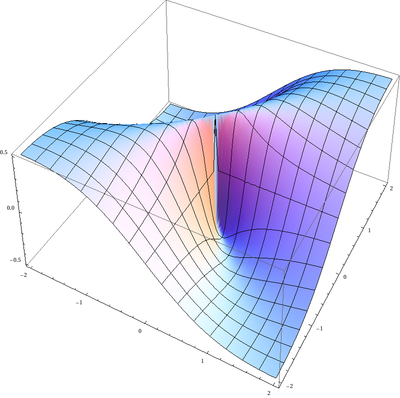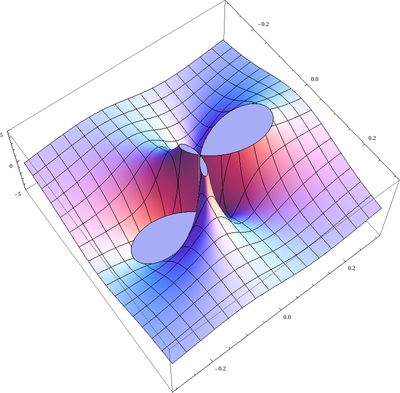6760, Math 21a, Fall 2009
Exhibits page Math 21a 2009, Multivariable Calculus
Discontinuous function for which partial derivatives exist
Office: SciCtr 434

## Discontinuous function for which partial derivatives existThe function fThe partial derivative fx

Continuity can be a bitch (*). You have seen already examples like here, where a function can be discontinuous even so all directional derivatives exist. Hairer and Wanner: Analysis by its history, Springer 2008, page 304. mention that for the following discontinuous discontinuous function all partial derivatives exist at (0,0). This is obvious since for x=0 as well as for y=0 the function is zero.
```f(x,y) = xy/(x2+y2)
```
But note that the function fx is not continuous everywhere. It is the function
```g(x,y)  = (-(x2y) + y3)/(x2 + y2)2
```

 If a function f(x,y) has continuous partial derivatives everywhere in the plane, then it is also continuous everywhere in the plane.

The above example shows that this statement is not true if one looks only at a single point.

(*) "Bitch" has been reappropriated to have positive meanings in some contexts.